Thursday 20th February 2020### You are in:Classroom Keystage 1·Maths·@school Assessment Tests·Calculations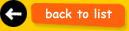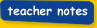Mathematics KS1Sum 1Look at the following sums and work out what numbers need to appear to add up to the answer.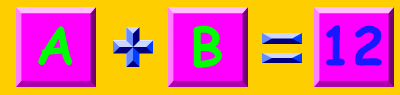A = 971101213----------------------------B = 4567121510----------------------------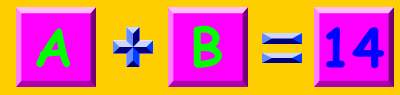A =  871101213----------------------------B =  4579121510----------------------------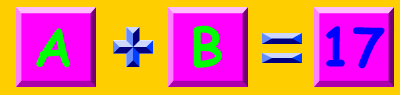A =  871111314----------------------------B =  579121518----------------------------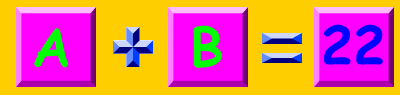A =  45891215----------------------------B =  589121518----------------------------